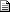Maths - Reflection - open forum discussion

 By: nobody ( Nobody/Anonymous )mirroring transformations   2003-10-20 23:43 i'm trying to write a tool to mirror the transformation of an object across an arbitrary plane, and was wondering if anyone could help me out. i'm only somewhat familiar with vector maths, so go easy. its application is for an animation package, and basically it will work like this. the user specifies object A to mirror, and object B to act as the plane to mirror across. then using the yz plane from object B, the translation and rotation are then mirrored and re-applied to object A. thanks in advance.
 By: martinbaker ( Martin Baker )RE: mirroring transformations   2003-10-21 08:36 I've been intending to do some stuff about reflections because its related to rotations. So I'll try to give a quick answer now, and if no one else does I'll try to have a fuller answer later. I assume your objects are defined in terms of 3D points, so the problem comes down to reflecting a number of 3D points across a plane. One way I can see to do this graphically, is as follows, if: P1 = a point you want to reflect (input) P2 = the reflected point (output) PN = a point 'P' on the plane such that, a vector from P1 to PN is normal to the plane Therefore a vector from PN to P1 = the inverse of (minus) a vector from PN to P2, which gives: P1 - PN = -(P2 - PN) Rearranging gives: P2 = 2*PN - P1 So the problem is calculating PN for each P1, I think this can be done using the methods on this page. https://www.euclideanspace.com/maths/geometry/elements/plane/lineOnPlane/ I think what you want is a matrix to transform the points on your objects, I will try to work it out from the above, but there are some special cases which it is easy to find, for example: Reflection in yz -1 0 0 0 1 0 0 0 1 Reflection in xz 1 0 0 0 -1 0 0 0 1 Reflection in xy 1 0 0 0 1 0 0 0 -1 Ill try to do some work on the general case, Martin
 By: nobody ( Nobody/Anonymous )RE: mirroring transformations   2003-10-21 15:53 yeah its not quite as simple as reflecting just points. as my object is essentially a single body, with rotation as well as translation properties. so i need to reflect the translation of its origin, but also reflect the rotation of its local axes. the problem of course is that the reflection won't be a true reflection, because it has to remain a right handed system. does that make any sense? it just has to be a visual reflection of the rotation, as the handedness cannot change.
 By: martinbaker ( Martin Baker )RE: mirroring transformations   2003-10-22 08:32 I think I understand but I'm not sure? If you do a reflection (say by inverting one axis) then the component of any vector which is normal to the mirror plane will be inverted. Rotation can be represented by a vector along the axis of rotation and this would be inverted in the same way. Since the rotation vector is inverted and the coordinate vectors are inverted, they will cancel out and the object will appear to rotate in the same direction. I have started to do some work on this subject here: https://www.euclideanspace.com/maths/geometry/affine/reflection/ but it may take some time to complete this. MartinClifford Algebra to Geometric Calculus: A Unified Language for Mathematics and Physics (Fundamental Theories of Physics). This book is intended for mathematicians and physicists rather than programmers, it is very theoretical. It covers the algebra and calculus of multivectors of any dimension and is not specific to 3D modelling.